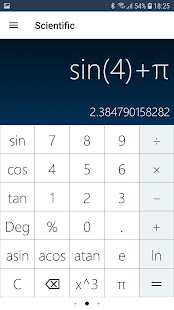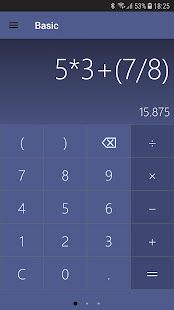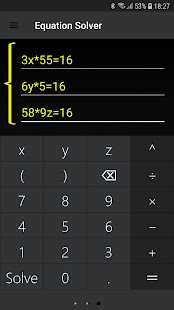# Math Calculator with Equation Solver

## Perform simple & complex mathematical calculations - Best Calculator for Android

3.70 EDUCATIONLooking for a substitute and better Math Calculator for your Android smartphone? Use this amazing Android Calculator app that is extremely easy to use and supports not only simple mathematical calculations but can also help you with complex scientific mathematical calculations.

Calculator app includes basic addition, subtraction, multiplication, division with its basic calculator functions and can also solve scientific mathematical problems with the scientific calculator function. Besides these, the Calculator can also help you with an equation solver that can solve equations easily. This free calculator app is a great replacement for the native android calculator and is extremely powerful and pocket-friendly. On this calculator app, you can find multi-line equation solver that can solve equations up to 3 lines. This is a very useful feature for mathematics & statistic students.

CALCULATOR FEATURES

Calculator is a must have app for all mathematics & statistics students, professionals or even for regular people for their day to day calculations. Here are features of the calculator app that make it among the best calculator apps for android -
- Basic Calculator to support basic math functions like Addition, Subtraction, Multiplication, Division, etc.
- Scientific Calculation Support to support Trigonometric calculation, anti-trigonometric calculation, power calculation, logarithmic calculation, square root calculation, cube root calculation, factorial calculation, π calculation and so on.
- Multi-line equation solver to solve complex mathematical equations in 3 lines.
- Customize the color theme as per your choice.
- Extremely easy to use and get started.

All these features on the Calculator app are free to use. So, what are you waiting for? If you are looking for the best and pocket-friendly calculator app to replace the native calculator on your Android phone, start using this “Calculator” app now.

We are constantly working hard on making the “Calculator” app better and more useful for you. We need your constant support to get going. Please feel free to email us for any queries/suggestions/problems or if you just want to say hi. We would love to hear from you. If you have enjoyed any feature of the “Calculator” app, do not forget to rate us on play store and share among your friends.

Bug fixes.

### Other versions

1.7.3 released on 15 August 2020 (430 days ago)
1.7.0 released on 30 July 2020 (446 days ago)
1.6.3 released on 29 March 2020 (569 days ago)

### Details and Recent Ratings

Size: 22 MB
Version: 1.7.4 by CalCon Mobile
Updated: 26 August 2020 (419 days ago)
Released: 11 December 2018
Installations: more than 50 000
5 Stars: 127
4 Stars: 25
3 Stars: 11
2 Stars: 6
1 Star: 56

### Similar apps

Android Game Categories
Android App Categories